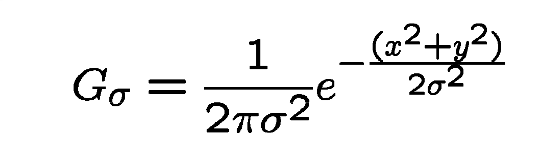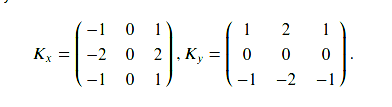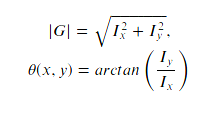GFG App
Open AppBrowser
Continue

# Implement Canny Edge Detector in Python using OpenCV

In this article, we will learn the working of the popular Canny edge detection algorithm developed by John F. Canny in 1986. Usually, in Matlab and OpenCV we use the canny edge detection for many popular tasks in edge detection such as lane detection, sketching, border removal, now we will learn the internal working and implementation of this algorithm from scratch.

## Theoretical Understanding

The basic steps involved in this algorithm are:

• Noise reduction using Gaussian filter

• Gradient calculation along the horizontal and vertical axis

• Non-Maximum suppression of false edges

• Double thresholding for segregating strong and weak edges

• Edge tracking by hysteresis

Now let us understand these concepts in detail:

#### 1. Noise reduction using Gaussian filter

This step is of utmost importance in the Canny edge detection. It uses a Gaussian filter for the removal of noise from the image, it is because this noise can be assumed as edges due to sudden intensity change by the edge detector. The sum of the elements in the Gaussian kernel is 1, so, the kernel should be normalized before applying as convolution to the image. In this tutorial, we will use a kernel of size 5 X 5 and sigma = 1.4, which will blur the image and remove the noise from it. The equation for Gaussian filter kernel isWhen the image is smoothed, the derivatives Ix and Iy are calculated w.r.t x and y axis. It can be implemented by using the Sobel-Feldman kernels convolution with image as given:Sobel Kernels

after applying these kernel we can use the gradient magnitudes and the angle to further process this step. The magnitude and angle can be calculated as#### 3. Non-Maximum Suppression

This step aims at reducing the duplicate merging pixels along the edges to make them uneven. For each pixel find two neighbors in the positive and negative gradient directions, supposing that each neighbor occupies the angle of pi /4, and 0 is the direction straight to the right. If the magnitude of the current pixel is greater than the magnitude of the neighbors, nothing changes, otherwise, the magnitude of the current pixel is set to zero.

#### 4. Double Thresholding

The gradient magnitudes are compared with two specified threshold values, the first one is lower than the second. The gradients that are smaller than the low threshold value are suppressed, the gradients higher than the high threshold value are marked as strong ones and the corresponding pixels are included in the final edge map. All the rest gradients are marked as weak ones and pixels corresponding to these gradients are considered in the next step.

#### 5. Edge Tracking using Hysteresis

Since a weak edge pixel caused by true edges will be connected to a strong edge pixel, pixel W with weak gradient is marked as edge and included in the final edge map if and only if it is involved in the same connected component as some pixel S with strong gradient. In other words, there should be a chain of neighbor weak pixels connecting W and S (the neighbors are 8 pixels around the considered one). We will make up and implement an algorithm that finds all the connected components of the gradient map considering each pixel only once. After that, you can decide which pixels will be included in the final edge map.
Below is the implementation.

## Python3

 `import` `numpy as np ` `import` `os ` `import` `cv2 ` `import` `matplotlib.pyplot as plt ` ` `  `  `  `# defining the canny detector function ` `  `  `# here weak_th and strong_th are thresholds for ` `# double thresholding step ` `def` `Canny_detector(img, weak_th ``=` `None``, strong_th ``=` `None``): ` `     `  `    ``# conversion of image to grayscale ` `    ``img ``=` `cv2.cvtColor(img, cv2.COLOR_BGR2GRAY) ` `      `  `    ``# Noise reduction step ` `    ``img ``=` `cv2.GaussianBlur(img, (``5``, ``5``), ``1.4``) ` `      `  `    ``# Calculating the gradients ` `    ``gx ``=` `cv2.Sobel(np.float32(img), cv2.CV_64F, ``1``, ``0``, ``3``) ` `    ``gy ``=` `cv2.Sobel(np.float32(img), cv2.CV_64F, ``0``, ``1``, ``3``) ` `     `  `    ``# Conversion of Cartesian coordinates to polar  ` `    ``mag, ang ``=` `cv2.cartToPolar(gx, gy, angleInDegrees ``=` `True``) ` `      `  `    ``# setting the minimum and maximum thresholds  ` `    ``# for double thresholding ` `    ``mag_max ``=` `np.``max``(mag) ` `    ``if` `not` `weak_th:weak_th ``=` `mag_max ``*` `0.1` `    ``if` `not` `strong_th:strong_th ``=` `mag_max ``*` `0.5` `     `  `    ``# getting the dimensions of the input image   ` `    ``height, width ``=` `img.shape ` `      `  `    ``# Looping through every pixel of the grayscale  ` `    ``# image ` `    ``for` `i_x ``in` `range``(width): ` `        ``for` `i_y ``in` `range``(height): ` `              `  `            ``grad_ang ``=` `ang[i_y, i_x] ` `            ``grad_ang ``=` `abs``(grad_ang``-``180``) ``if` `abs``(grad_ang)>``180` `else` `abs``(grad_ang) ` `              `  `            ``# selecting the neighbours of the target pixel ` `            ``# according to the gradient direction ` `            ``# In the x axis direction ` `            ``if` `grad_ang<``=` `22.5``: ` `                ``neighb_1_x, neighb_1_y ``=` `i_x``-``1``, i_y ` `                ``neighb_2_x, neighb_2_y ``=` `i_x ``+` `1``, i_y ` `             `  `            ``# top right (diagonal-1) direction ` `            ``elif` `grad_ang>``22.5` `and` `grad_ang<``=``(``22.5` `+` `45``): ` `                ``neighb_1_x, neighb_1_y ``=` `i_x``-``1``, i_y``-``1` `                ``neighb_2_x, neighb_2_y ``=` `i_x ``+` `1``, i_y ``+` `1` `             `  `            ``# In y-axis direction ` `            ``elif` `grad_ang>(``22.5` `+` `45``) ``and` `grad_ang<``=``(``22.5` `+` `90``): ` `                ``neighb_1_x, neighb_1_y ``=` `i_x, i_y``-``1` `                ``neighb_2_x, neighb_2_y ``=` `i_x, i_y ``+` `1` `             `  `            ``# top left (diagonal-2) direction ` `            ``elif` `grad_ang>(``22.5` `+` `90``) ``and` `grad_ang<``=``(``22.5` `+` `135``): ` `                ``neighb_1_x, neighb_1_y ``=` `i_x``-``1``, i_y ``+` `1` `                ``neighb_2_x, neighb_2_y ``=` `i_x ``+` `1``, i_y``-``1` `             `  `            ``# Now it restarts the cycle ` `            ``elif` `grad_ang>(``22.5` `+` `135``) ``and` `grad_ang<``=``(``22.5` `+` `180``): ` `                ``neighb_1_x, neighb_1_y ``=` `i_x``-``1``, i_y ` `                ``neighb_2_x, neighb_2_y ``=` `i_x ``+` `1``, i_y ` `              `  `            ``# Non-maximum suppression step ` `            ``if` `width>neighb_1_x>``=` `0` `and` `height>neighb_1_y>``=` `0``: ` `                ``if` `mag[i_y, i_x]neighb_2_x>``=` `0` `and` `height>neighb_2_y>``=` `0``: ` `                ``if` `mag[i_y, i_x]grad_mag>``=` `weak_th: ` `                ``ids[i_y, i_x]``=` `1` `            ``else``: ` `                ``ids[i_y, i_x]``=` `2` `      `  `      `  `    ``# finally returning the magnitude of ` `    ``# gradients of edges ` `    ``return` `mag ` `  `  `frame ``=` `cv2.imread(``'food.jpeg'``) ` ` `  `# calling the designed function for ` `# finding edges ` `canny_img ``=` `Canny_detector(frame) ` `  `  `# Displaying the input and output image   ` `plt.figure() ` `f, plots ``=` `plt.subplots(``2``, ``1``)  ` `plots[``0``].imshow(frame) ` `plots[``1``].imshow(canny_img) `Input imageOutput image

My Personal Notes arrow_drop_up